##### ASVAB AFQT For Dummies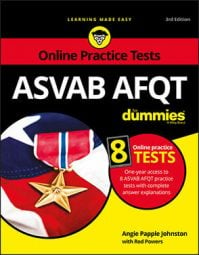The least common multiple (LCM) of two or more numbers is the smallest number (not zero) that's a multiple of both or all the numbers. Finding the LCM is a useful technique for solving many problems on the ASVAB AFQT math subtests—especially those involving fractions.

One way to find the LCM is to list the multiples of each number, one at a time, until you find the smallest multiple that's common to all the numbers.

Try the following example: find the LCM of 45 and 50.

• Multiples of 45: 45, 90, 135, 180, 225, 270, 315, 360, 405, 450
• Multiples of 50: 50, 100, 150, 200, 250, 300, 350, 400, 450
The LCM of 45 and 50 is 450.

That's rather cumbersome, isn't it? Wouldn't it be great if you had an easier way? Fortunately, you do: An easier way to find the LCM is first to list the prime factors of each number:

• The prime factors for 45 are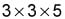• The prime factors for 50 are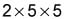Then multiply each factor the greatest number of times it occurs in either number. If the same factor occurs more than once in both numbers, you multiply the factor the greatest number of times it occurs. For example, 5 occurs as a prime factor of both 45 (where it occurs once) and 50 (where it occurs twice); the two occurrences in the factorization of 50 beat the single occurrence in the factorization of 45. The number 3 occurs two times, 5 occurs two times, and 2 occurs once, so you have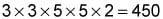Here's one more example: what is the least common multiple of 5, 27, and 30?

List the prime factors of each number:

• Prime factors of 5: 5
• Prime factors of 27: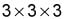• Prime factors of 30: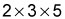The number 3 occurs a maximum of three times, 5 occurs a maximum of one time, and 2 occurs a maximum of one time: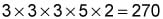Check your answer by seeing whether 5, 27, and 30 can all divide evenly into 270.